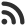Feed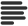Articles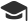Tutorials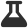Lab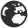CompaniesLeaderboard
 VK Programmer and Software Engineer at Tata Consultancy Services Jan. 8, 2021, 7:55 p.m. ⋅ 975 views

# Program Code - calculate the absolute difference between the sums of its diagonals.

Given a square matrix, calculate the absolute difference between the sums of its diagonals.

For example, the square matrix  is shown below:

``````1 2 3
4 5 6
9 8 9
``````

The left-to-right diagonal = . The right to left diagonal = . Their absolute difference is .

Function description

Complete the  function in the editor below.

diagonalDifference takes the following parameter:

• int arr[n][m]: an array of integers

Return

• int: the absolute diagonal difference

Input Format

The first line contains a single integer, , the number of rows and columns in the square matrix .
Each of the next  lines describes a row, , and consists of  space-separated integers .

Constraints

•

Output Format

Return the absolute difference between the sums of the matrix's two diagonals as a single integer.

Sample Input

``````3
11 2 4
4 5 6
10 8 -12
``````

Sample Output

``````15
``````

Explanation

The primary diagonal is:

``````11
5
-12
``````

Sum across the primary diagonal: 11 + 5 - 12 = 4

The secondary diagonal is:

``````     4
5
10
``````

Sum across the secondary diagonal: 4 + 5 + 10 = 19
Difference: |4 - 19| = 15

Note: |x| is the absolute value of x

Solution Code :-

 number = int(input("Enter no of rows:- ")) arr = [[int(input()) for j in range(0,number)] for i in range(0,number)] def diagonalDifference(arr):     #Write your code here     arr_length = len(arr)     forword_diagonal = 0     backword_diagonal = 0     reverse_count = arr_length-1     for idx in range(0,arr_length):         forword_diagonal+=arr[idx][idx]         backword_diagonal+=arr[idx][reverse_count]         reverse_count-=1     return(abs(forword_diagonal -backword_diagonal )) print(diagonalDifference(arr))

Events

Nov. 28, 2018, 5:30 p.m.

Python from zero to hero

place Delhi

Aug. 13, 2018, 5:30 p.m.

Python from zero to hero

place Bangalore ( HackersFriend office BTM Layout)